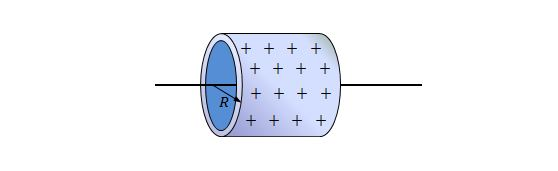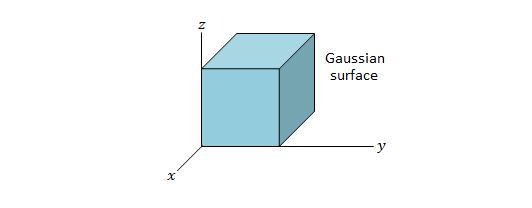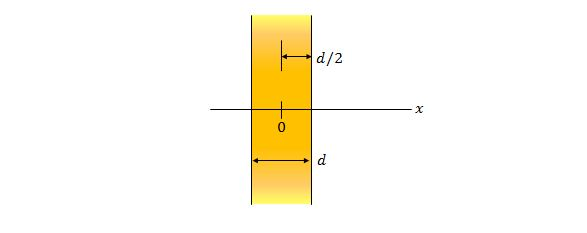Electricity and Magnetism

# Problem solving - Flux and Gauss' lawConsider an infinitely long, very thin metal tube with radius $R=2.90\text{ cm}.$ The above figure shows a section of it. If the linear charge density of the cylinder is $\lambda=1.50 \times 10^{-8} \text{ C/m},$ what is the approximate magnitude of the electric field at radial distance $r=2R?$

The value of the permittivity constant is $\varepsilon_0=8.85 \times 10^{-12} \text{ C}^2\text{/N}\cdot\text{m}^2.$

Consider a solid sphere of radius $a=2.30\text{ cm},$ which has a net uniform charge of $q=+4.50\text{ fC}.$ What is the approximate magnitude of the electric field at radial distances (a) $r=a/2$ and (b) $r=a ?$

The value of electrostatic constant is $\displaystyle k=\frac{1}{4\pi\varepsilon_0}=8.99 \times 10^9 \text{ N}\cdot\text{m}^2\text{/C}^2.$Consider a metal rod with radius of $R_1=1.20\text{ mm}$ and length $L=12.00\text{ m},$ which is inside a very thin coaxial metal cylinder with radius of $R_2=10.0 R_1$ and length $L,$ as shown in the above figure. If the net charge on the rod and on the cylinder is $Q_1=+3.40 \times 10^{-12}\text{ C}$ and $Q_2=-2.0 Q_1,$ respectively, what is the approximate magnitude of the electric field at radial distance $r=2.0 \times R_2,$ assuming that the charge density of both the rod and the cylinder are uniform?

The value of the permittivity constant is $\varepsilon_0=8.85 \times 10^{-12} \text{ C}^2\text{/N}\cdot\text{m}^2.$Consider a Gaussian surface in the shape of a cube with edge length $2.40\text{ m},$ as shown in the above figure. If the electric field in which the Gaussian surface lies can be expressed as $\vec{E}=(2.00x+3.00)\hat{i}+5.00\hat{j}+6.00\hat{k} \text{ (N/C)},$ where $x$ is in meters, what is the approximate net charge contained by the Gaussian surface?

The value of the permittivity constant is $\varepsilon_0=8.85 \times 10^{-12} \text{ C}^2\text{/N}\cdot\text{m}^2.$Consider an infinitely large non-conducting plate of thickness $d=9.20\text{ mm}.$ The above figure shows a cross section of the plate, where the origin of the $x$-axis is at the plate's center. If the plate has a uniform charge density of $\rho=5.80\text{ fC/m}^3,$ what is the magnitude of the electric field at the $x$-coordinate of (a) $4.60\text{ mm}$ and (b) $27.0 \text{ mm}?$

The value of the permittivity constant is $\varepsilon_0=8.85 \times 10^{-12} \text{ C}^2\text{/N}\cdot\text{m}^2.$

×